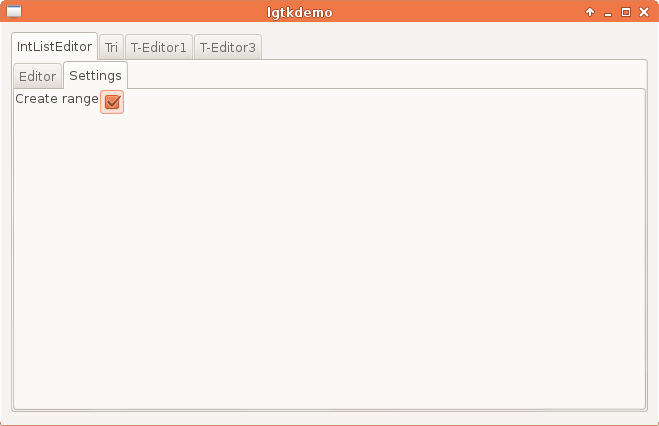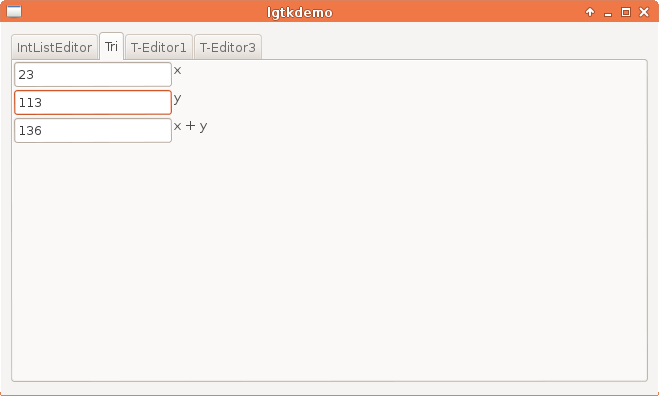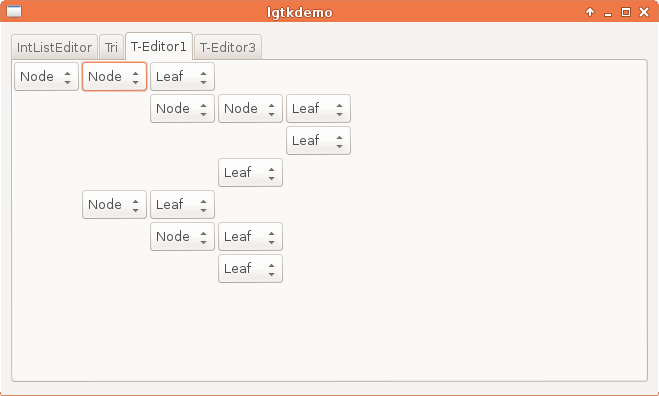# 1 Introduction

Most Haskellers would like to use a mature FRP-based GUI.
I think FRP may not be the best tool for special user interfaces, like interfaces which consists of buttons, checkboxes, combo boxes, text entries and menus only.
I present a prototype lens-based model which fits better these user interfaces.

# 2 Demo applications

First I would like to show some screenshots of a demo application. It has separate demos in its tabs.
While reading the features of the demos, think about how much lines would you need to code these demos in your favourite programming language and GUI framework.

You can also try the demo application, the needed steps are:

``````cabal install gtk2hs-buildtools
cabal install lgtk
lgtkdemo``````

## 2.1 Int list editorInt list editor

Features:

• The number of entries may vary from 0 to 15.
• When you add an item, the last item is copied (with its selection state).
The value of the added item is either the same, or the previous value plus one.
This is a dynamic behaviour which can be changed on the settings tab (see next screenshot).
• Each item can be deleted or copied individually.
• The sum of the selected items are shown.
• Sorting: the selections move around with the items when sorted
• `DeleteAll` and `DelSel` buttons shows how many items would be deleted.
• Selections
• positive or even items may be selected at once
• invert selection
• delete / copy selected items
• add (+1) / (-1) to selected items
• Undo / redo
• selection changes are not recorded (this is a feature)
• State save (if you quit and restart, it remembers its state)
• Each button is active only when it has an effect.
• The application is fool-proof.Int list editor settingsx + y = z editor

Features:

• The last item is always the sum of the first two items.
• The last edited entry changes.

## 2.3 Binary tree shape editorBinary tree shape editor

Features:

• You can edit the shape of a binary tree.
• Edited subtrees are remembered, so if you select `Leaf` and `Node` again, you get back the previous state.

The same editor with checkboxes:Binary tree shape editor with checkboxes

## 2.4 LOC

The Int list editor is less than 70 lines in LGtk.
I did not count the generic parts, like a generic undo-redo state transformation.

The addition relation editor is 20 lines in LGtk.

The binary tree shape editor is 10 lines in LGtk.

# 3 LGtk Overview

The lens-based Gtk interface has the following ingredients:

• expandable state
• lens-based Gtk API parametrised by a monad

The data type of monadic lenses is:

``````newtype MLens m a b
= MLens (a -> m (b, b -> m a))``````

Side-effect free lenses have type either

``type Lens a b = MLens Identity a b``

or

``type Lens a b = forall m . Monad m => MLens m a b``

Monadic lenses are handy because they contain the well-known references, for example IO references:

``type Ref m a = MLens m () a``

In this way references can easily be composed with lenses.
For example, if

``r :: Ref m (a,b)``

then

``fstLens . r :: Ref m a``

Note that LGtk use lots of impure lenses (lenses which do not fulfil the lens laws).
Using lenses which do not fulfil the lens laws are safe, but one should take extra care when doing program transformations or reasoning about code with impure lenses.

## 3.2 Expandable state

### 3.2.1 The `NewRef` class

Monads with reference creation can be given the `NewRef` class instance:

``````class (Monad m) => NewRef m where
newRef :: a -> m (Ref m a)``````
``instance NewRef IO``

### 3.2.2 The `ExtRef` class

Suppose that we would like to extend a reference with a hidden state.
You can think of this operation as backward application of a lens to a reference.

This operation can be done in the `NewRef` class:

``````class NewRef m => ExtRef m where
extRef :: Ref m b -> MLens m a b -> a -> m (Ref m a)``````

Explanation of `extRef`:

Suppose that `k` is a pure lens, and

``s <- extRef r k a0``

Then following laws should hold:

• `(k . s)` behaves exactly as `r`.
• The initial value of `s` is the result of `(readRef r >>= setL k a0)`.

Moreover, `(extRef r k a0)` should not change the value of `r`.

It is better to explain this on concrete examples.
I implemented the following tests:

``````newRefTest = runTest \$ do
r <- newRef 3             -- create a new reference with initial value 3
r ==> 3                   -- reading the reference should give 3``````
``````writeRefTest = runTest \$ do
r <- newRef 3
r ==> 3                   -- value before write
writeRef r 4
r ==> 4                   -- value after write``````
``````extRefTest = runTest \$ do
r <- newRef \$ Just 3      -- r is a (Maybe Int) reference
q <- extRef r maybeLens (False, 0)
-- we extend r's state; maybeLens :: Lens m (Bool, a) (Maybe a)
let q1 = fstLens . q      -- lens composition
q2 = sndLens . q
r ==> Just 3              -- r is still (Just 3)
q ==> (True, 3)           -- q is (True, 3)
writeRef r Nothing
r ==> Nothing
q ==> (False, 3)          -- q still holds the value 3
q1 ==> False
writeRef q1 True
r ==> Just 3              -- we have got back (Just 3)
writeRef q2 1
r ==> Just 1``````
``````joinTest = runTest \$ do
r2 <- newRef 5
r1 <- newRef 3
rr <- newRef r1
r1 ==> 3
let r = joinLens rr       -- lenses can be joined, which is really handy for dynamic interfaces
r ==> 3
writeRef r1 4
r ==> 4
writeRef rr r2            -- switching to another source
r ==> 5
writeRef r1 4
r ==> 5
writeRef r2 14
r ==> 14``````

With the help of `ExtRef`, one can define undo-redo state transformation (I don’t give more details now):

``````undoTr
:: ExtRef m =>
(a -> a -> Bool)     -- equality on state
-> Ref m a              -- reference of state
-> m ( m (Maybe (m ())) -- undo action, Nothing if it has no sense
, m (Maybe (m ())) -- redo action, Nothing if it has no sense
)``````

## 3.3 Lens-based Gtk API

Now, the Gtk API is that simple (this is a prototype without styling):

``runI :: I IO -> IO ()     -- we need only one rendering function``
``````data I m
= Label (m String)              -- ^ label
| Button { label  :: m String
, action :: m (Maybe (m ()))     -- ^ when the @Maybe@ value is @Nothing@, the button is inactive
}                      -- ^ button
| Checkbox (Ref m Bool)         -- ^ checkbox
| Entry (Ref m String)          -- ^ entry field
-- ...
| List ListLayout [I m]         -- ^ group interfaces into row or column
| forall a . Eq a
=> Cell { underlying_value   :: m a
, dynamic_interface  :: a -> I m
}                       -- ^ dynamic interface
| Action (m (I m))              -- ^ do an action before giving the interface``````
``````data ListLayout
= Horizontal | Vertical``````

In the actual interface I use also free monads to speed up the implementation.